# Double Your Pleasure – Fun with Shifty Digits

You’re in for a guest-blogging treat today. But first…

What is the relationship between the number 1947 and 7194? Look closely and you’ll see that they’re identical, except the digit 7 has been moved from the back to the front.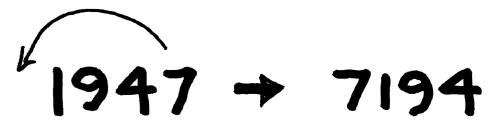A minor textual change has a big effect in numeric space. MATLAB Central hero John D’Errico has something to say about this relationship in his guest post below. But before we get to that I want to make a few comments. The first is to thank John for sending me this post! He sent me a Live Script (the MATLAB file type with the suffix MLX), and I converted it to a WordPress blog post here. That leads me to my second comment: how would you like to be a guest blogger on this site? If you have a Live Script on an interesting topic and you’d like to publish it, send it to me (gulley@mathworks.com). We can work together and, who knows? You might end up with a byline on MATLAB Central.

And now for you treat. Watch John as he solves a fiendish problem with deft strokes. Although I would ask you, once you understand the problem statement, to try solving it yourself before you get to the end. I bet you won’t do as well as John. I couldn’t, anyway…

by John D’Errico

What positive integer doubles when its last digit (thus the units digit) is moved to become the first or highest order digit? Assume a decimal representation for the digits. In fact, this is a rather well known problem. For example, you can find it discussed on YouTube.

The question is also interesting when posed in binary. Does a solution exist in binary? That is, does there exist a binary number, perhaps 10101011101, such that if we shift that unit bit to the left end of the number, the number will exactly double?

Alas, we can prove such a solution can never exist, at least, not in binary. The proof is simple. Imagine a solution did exist, composed of exactly N binary bits. The shift we have described does not increase the number of bits in the number. It is merely a pure transposition of the existing bits.

However, when happens when you multiply ANY binary number by 2? As long as the number is non-zero, then if you multiply by 2, you always increase the number of bits in the number by 1. Therefore our goal is impossible for any binary number. It is possible for this to happen if the number is written in base 3 or above. We can investigate that possibility later, but first how does this work in base 10?

Back to base 10, We have postulated that some decimal number exists such that a cyclic move of the lowest order decimal digits from the units place to now become the highest order digit, will then double the number. Again, this does not change the number of decimal digits in the number.

We can try some simple examples, just to see how it might work. For example, consider the number 102. The transformation here would have us create the new number 210, made by moving the 2 from the lowest order digit , into the highest order digit. However, this does not double the number.

2*102 ~= 210

ans = logical
1



We might try other numbers, perhaps 12345, but doubling that number will not yield 51234. In fact, I’ll assert that a brute force solution to this problem will take some effort. We need to use mathematics to solve our problem.

Our goal is to find a decimal number of the form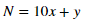In that expression, y is a single digit integer, x an integer of some arbitrary order. Combined, they must satisfy the relation: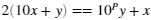In that relation, P is the number of decimal digits in the number N. Then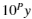simply acts as a shift for the units digit by P places to the left. We need to search for the smallest such solution, in case multiple solutions do exist.

One important question to ask is if the units digit can ever be 0? What happens if y is zero above? Then the governing equation reduces to the rather simple case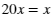with the solution x == 0. So the only possible solution to our problem if the number in question has a zero units digit is very boring N == 0. Since we have already required N must be a positive integer, that case is impossible. Therefore y must come from the set [1:9]. With some additional effort, we might be able to be more complete, but 1:9 is a good start. Return now to the governing equation of this problem, now isolating x and y on opposite sides of the equality.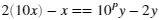or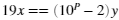If the variables in this equation are integers, then the Fundamental Theorem of Arithmetic tells us that 19 (as a prime number) must either divide y, or it must divide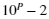. However, we just stated that y must come from the set 1:9. We can now conclude 19 must divide.

What, therefore, is the smallest power of P, such that 19 divides 10^P-2? (Note: the SYM command used below is from the Symbolic Math Toolbox)

P = find(mod(sym(10).^(1:100) - 2,19) == 0)

P =

17    35    53    71    89


diff(P)

ans =

18    18    18    18



This is useful. The smallest possible power P such thathas 19 as an integer divisor is 17. It appears that every 18 powers of 10 after that point also have the same property, but we will first be interested in the case where P=17, as if a smallest solution exists, it may have 18 decimal digits. I did suggest before that brute force would be a difficult way to solve this problem.

At least, if a solution does exist, we can do the computations using perhaps int64. Double precision will fail us though, since doubles tap out around 16 decimal digits, before thy can no longer represent an integer exactly. The headroom exists in int64 however, since

intmax('int64')

ans = int64
9223372036854775807


That is a number with 19 decimal digits. uint64 would allow us to push slightly higher, but int64 is entirely sufficient.

Now, suppose we had a units digit from the set 1:9?

unitsdigit = int64(1:9)';
P = int64(17);


Now we have sufficient information to possibly solve for x.

x = (int64(10)^P - 2)*unitsdigit/int64(19);
N = 10*x + unitsdigit

N = 9×1 int64 column vector

52631578947368421
105263157894736842
157894736842105263
210526315789473684
263157894736842105
315789473684210526
368421052631578947
421052631578947368
473684210526315789



Those are the potential values of x. We can see if they worked. That is, if we double N, then we must have the same result, as if we transposed the units digit to now become the highest order digit.

[2*N,10^P*unitsdigit + x]

ans = 9×2 int64 matrix

105263157894736842   105263157894736842
210526315789473684   210526315789473684
315789473684210526   315789473684210526
421052631578947368   421052631578947368
526315789473684210   526315789473684210
631578947368421052   631578947368421052
736842105263157894   736842105263157894
842105263157894736   842105263157894736
947368421052631578   947368421052631578



The first case, with y == 1, fails the test, because we see an extra 0 inserted as the second highest order digit. However, that leaves the second case, with x == 2, as the minimal solution. This must be the smallest number that has the desired property.

N(2)

ans = int64
105263157894736842

2*(10*x(2) + unitsdigit(2)) == 10^P*unitsdigit(2) + x(2)

ans = logical
1



There were 8 other solutions found, all of which were valid, except they are not minimal. Beyond that point, the next solution would have 36 decimal digits, well beyond the limits of even uint64.

Again, be careful in this, as these computations would have failed in double precision arithmetic.

|